# Tag: Norton

An integral $n$-dimensional lattice $L$ is the set of all integral linear combinations
$L = \mathbb{Z} \lambda_1 \oplus \dots \oplus \mathbb{Z} \lambda_n$
of base vectors $\{ \lambda_1,\dots,\lambda_n \}$ of $\mathbb{R}^n$, equipped with the usual (positive definite) inner product, satisfying
$(\lambda, \mu ) \in \mathbb{Z} \quad \text{for all \lambda,\mu \in \mathbb{Z}.}$
But then, $L$ is contained in its dual lattice $L^* = Hom_{\mathbb{Z}}(L,\mathbb{Z})$, and if $L = L^*$ we say that $L$ is unimodular.

If all $(\lambda,\lambda) \in 2 \mathbb{Z}$, we say that $L$ is an even lattice. Even unimodular lattices (such as the $E_8$-lattice or the $24$ Niemeier lattices) are wonderful objects, but they can only live in dimensions $n$ which are multiples of $8$.

Just like the Conway group $Co_0 = .0$ is the group of rotations of the Leech lattice $\Lambda$, one might ask whether there is a very special lattice on which the Monster group $\mathbb{M}$ acts faithfully by rotations. If such a lattice exists, it must live in dimension at least $196883$.Simon Norton (1952-2019) – Photo Credit

A first hint of such a lattice is in Conway’s original paper A simple construction for the Fischer-Griess monster group (but not in the corresponding chapter 29 of SPLAG).

Conway writes that Simon Norton showed ‘by a very simple computations that does not even require knowledge of the conjugacy classes, that any $198883$-dimensional representation of the Monster must support an invariant algebra’, which, after adding an identity element $1$, we now know as the $196884$-dimensional Griess algebra.

Further, on page 529, Conway writes:

Norton has shown that the lattice $L$ spanned by vectors of the form $1,t,t \ast t’$, where $t$ and $t’$ are transposition vectors, is closed under the algebra multiplication and integral with respect to the doubled inner product $2(u,v)$. The dual quotient $L^*/L$ is cyclic of order some power of $4$, and we believe that in fact $L$ is unimodular.

Here, transposition vectors correspond to transpositions in $\mathbb{M}$, that is, elements of conjugacy class $2A$.

In his post, Adam considers the $196883$-dimensional lattice $L’ = L \cap 1^{\perp}$ (which has $\mathbb{M}$ as its rotation symmetry group), and asks for the minimal norm (squared) of a lattice point, which he believes is $448$, and for the number of minimal vectors in the lattice, which might be
$2639459181687194563957260000000 = 9723946114200918600 \times 27143910000$
the number of oriented arcs in the Monster graph.

Here, the Monster graph has as its vertices the elements of $\mathbb{M}$ in conjugacy class $2A$ (which has $9723946114200918600$ elements) and with an edge between two vertices if their product in $\mathbb{M}$ again belongs to class $2A$, so the valency of the graph must be $27143910000$, as explained in that old post the monster graph and McKay’s observation.

When I asked Adam whether he had more information about his lattice, he kindly informed me that Borcherds told him that the Norton lattice $L$ didn’t turn out to be unimodular after all, but that a unimodular lattice with monstrous symmetry had been constructed by Scott Carnahan in the paper A Self-Dual Integral Form of the Moonshine Module.Scott Carnahan – Photo Credit

The major steps (or better, the little bit of it I could grasp in this short time) in the construction of this unimodular $196884$-dimensional monstrous lattice might put a smile on your face if you are an affine scheme aficionado.

Already in his paper Vertex algebras, Kac-Moody algebras, and the Monster, Richard Borcherds described an integral form of any lattice vertex algebra. We’ll be interested in the lattice vertex algebra $V_{\Lambda}$ constructed from the Leech lattice $\Lambda$ and call its integral form $(V_{\Lambda})_{\mathbb{Z}}$.

One constructs the Moonshine module $V^{\sharp}$ from $V_{\Lambda}$ by a process called ‘cyclic orbifolding’, a generalisation of the original construction by Frenkel, Lepowsky and Meurman. In fact, there are now no less than 51 constructions of the moonshine module.

One starts with a fixed point free rotation $r_p$ of $\Lambda$ in $Co_0$ of prime order $p \in \{ 2,3,5,7,13 \}$, which one can lift to an automorphism $g_p$ of the vertex algebra $V_{\Lambda}$ of order $p$ giving an isomorphism $V_{\Lambda}/g_p \simeq V^{\sharp}$ of vertex operator algebras over $\mathbb{C}$.

For two distinct primes $p,p’ \in \{ 2,3,5,7,13 \}$ if $Co_0$ has an element of order $p.p’$ one can find one such $r_{pp’}$ such that $r_{pp’}^p=r_{p’}$ and $r_{pp’}^{p’}=r_p$, and one can lift $r_{pp’}$ to an automorphism $g_{pp’}$ of $V_{\Lambda}$ such that $V_{\Lambda}/g_{pp’} \simeq V_{\Lambda}$ as vertex operator algebras over $\mathbb{C}$.

Problem is that these lifts of automorphisms and the isomorphisms are not compatible with the integral form $(V_{\Lambda})_{\mathbb{Z}}$ of $V_{\Lambda}$, but ‘essentially’, they can be performed on
$(V_{\Lambda})_{\mathbb{Z}} \otimes_{\mathbb{Z}} \mathbb{Z}[\frac{1}{pp’},\zeta_{2pp’}]$
where $\zeta_{2pp’}$ is a primitive $2pp’$-th root of unity. These then give a $\mathbb{Z}[\tfrac{1}{pp’},\zeta_{2pp’}]$-form on $V^{\sharp}$.

Next, one uses a lot of subgroup information about $\mathbb{M}$ to prove that these $\mathbb{Z}[\tfrac{1}{pp’},\zeta_{2pp’}]$-forms of $V^{\sharp}$ have $\mathbb{M}$ as their automorphism group.

Then, using all his for different triples in $\{ 2,3,5,7,13 \}$ one can glue and use faithfully flat descent to get an integral form $V^{\sharp}_{\mathbb{Z}}$ of the moonshine module with monstrous symmetry and such that the inner product on $V^{\sharp}_{\mathbb{Z}}$ is positive definite.

Finally, one looks at the weight $2$ subspace of $V^{\sharp}_{\mathbb{Z}}$ which gives us our Carnahan’s $196884$-dimensional unimodular lattice with monstrous symmetry!

Beautiful as this is, I guess it will be a heck of a project to deduce even the simplest of facts about this wonderful lattice from running through this construction.

For example, what is the minimal length of vectors? What is the number of minimal length vectors? And so on. All info you might have is very welcome.

Whenever I visit someone’s YouTube or Twitter profile page, I hope to see an interesting banner image. Here’s the one from Richard Borcherds’ YouTube Channel.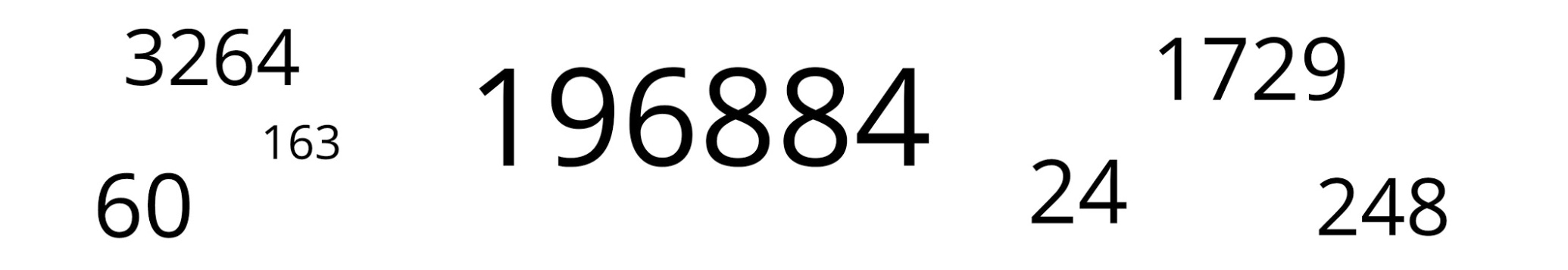Not too surprisingly for Borcherds, almost all of these numbers are related to the monster group or its moonshine.

Let’s try to decode them, in no particular order.

196884

John McKay’s observation $196884 = 1 + 196883$ was the start of the whole ‘monstrous moonshine’ industry. Here, $1$ and $196883$ are the dimensions of the two smallest irreducible representations of the monster simple group, and $196884$ is the first non-trivial coefficient in Klein’s j-function in number theory.

$196884$ is also the dimension of the space in which Robert Griess constructed the Monster, following Simon Norton’s lead that there should be an algebra structure on the monster-representation of that dimension. This algebra is now known as the Griess algebra.

Here’s a recent talk by Griess “My life and times with the sporadic simple groups” in which he tells about his construction of the monster (relevant part starting at 1:15:53 into the movie).

1729

1729 is the second (and most famous) taxicab number. A long time ago I did write a post about the classic Ramanujan-Hardy story the taxicab curve (note to self: try to tidy up the layout of some old posts!).

Recently, connections between Ramanujan’s observation and K3-surfaces were discovered. Emory University has an enticing press release about this: Mathematicians find ‘magic key’ to drive Ramanujan’s taxi-cab number. The paper itself is here.

“We’ve found that Ramanujan actually discovered a K3 surface more than 30 years before others started studying K3 surfaces and they were even named. It turns out that Ramanujan’s work anticipated deep structures that have become fundamental objects in arithmetic geometry, number theory and physics.”

Ken Ono

24

There’s no other number like $24$ responsible for the existence of sporadic simple groups.

24 is the length of the binary Golay code, with isomorphism group the sporadic Mathieu group $M_24$ and hence all of the other Mathieu-groups as subgroups.

24 is the dimension of the Leech lattice, with isomorphism group the Conway group $Co_0 = .0$ (dotto), giving us modulo its center the sporadic group $Co_1=.1$ and the other Conway groups $Co_2=.2, Co_3=.3$, and all other sporadics of the second generation in the happy family as subquotients (McL,HS,Suz and $HJ=J_2$)24 is the central charge of the Monster vertex algebra constructed by Frenkel, Lepowski and Meurman. Most experts believe that the Monster’s reason of existence is that it is the symmetry group of this vertex algebra. John Conway was one among few others hoping for a nicer explanation, as he said in this interview with Alex Ryba.

24 is also an important number in monstrous moonshine, see for example the post the defining property of 24. There’s a lot more to say on this, but I’ll save it for another day.

60

60 is, of course, the order of the smallest non-Abelian simple group, $A_5$, the rotation symmetry group of the icosahedron. $A_5$ is the symmetry group of choice for most viruses but not the Corona-virus.

3264

3264 is the correct solution to Steiner’s conic problem asking for the number of conics in $\mathbb{P}^2_{\mathbb{C}}$ tangent to five given conics in general position.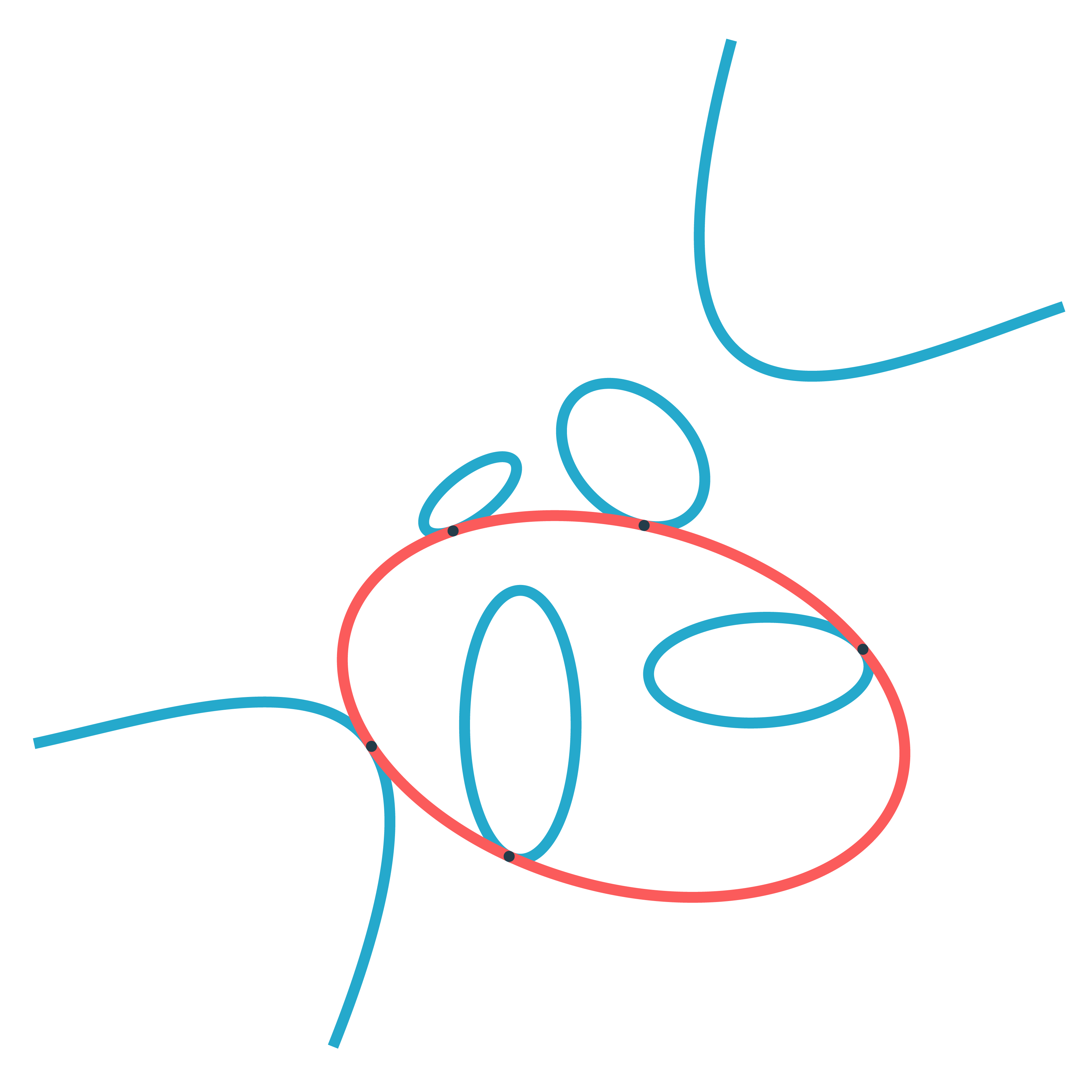Steiner himself claimed that there were $7776=6^5$ such conics, but realised later that he was wrong. The correct number was first given by Ernest de Jonquières in 1859, but a rigorous proof had to await the advent of modern intersection theory.

Eisenbud and Harris wrote a book on intersection theory in algebraic geometry, freely available online: 3264 and all that.

248

248 is the dimension of the exceptional simple Lie group $E_8$. $E_8$ is also connected to the monster group.

If you take two Fischer involutions in the monster (elements of conjugacy class 2A) and multiply them, the resulting element surprisingly belongs to one of just 9 conjugacy classes:

1A,2A,2B,3A,3C,4A,4B,5A or 6A

The orders of these elements are exactly the dimensions of the fundamental root for the extended $E_8$ Dynkin diagram.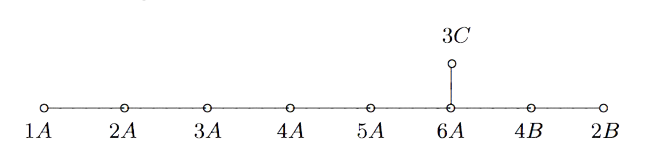This is yet another moonshine observation by John McKay and I wrote a couple of posts about it and about Duncan’s solution: the monster graph and McKay’s observation, and $E_8$ from moonshine groups.

163

163 is a remarkable number because of the ‘modular miracle’
$e^{\pi \sqrt{163}} = 262537412640768743.99999999999925…$
This is somewhat related to moonshine, or at least to Klein’s j-function, which by a result of Kronecker’s detects the classnumber of imaginary quadratic fields $\mathbb{Q}(\sqrt{-D})$ and produces integers if the classnumber is one (as is the case for $\mathbb{Q}(\sqrt{-163})$).

The details are in the post the miracle of 163, or in the paper by John Stillwell, Modular Miracles, The American Mathematical Monthly, 108 (2001) 70-76.

His description of the $j$-function (at 4:13 in the movie) is simply hilarious!

Borcherds connects $163$ to the monster moonshine via the $j$-function, but there’s another one.

The monster group has $194$ conjugacy classes and monstrous moonshine assigns a ‘moonshine function’ to each conjugacy class (the $j$-function is assigned to the identity element). However, these $194$ functions are not linearly independent and the space spanned by them has dimension exactly $163$.

SNORT, invented by Simon NORTon is a map-coloring game, similar to COL. Only, this time, neighbours may not be coloured differently.

SNORTgo, similar to COLgo, is SNORT played with go-stones on a go-board. That is, adjacent stones must have the same colour.

SNORT is a ‘hot’ game, meaning that each player is eager to move as most moves will improve your position. In COL players are reluctant to move, because a move limits your next moves.

For this reason, SNORT positions are much harder to evaluate, and one needs the full force of Conways’s ONAG.

Here’s a SNORTgo endgame. Who has a winning strategy?, and what is the first move in that strategy?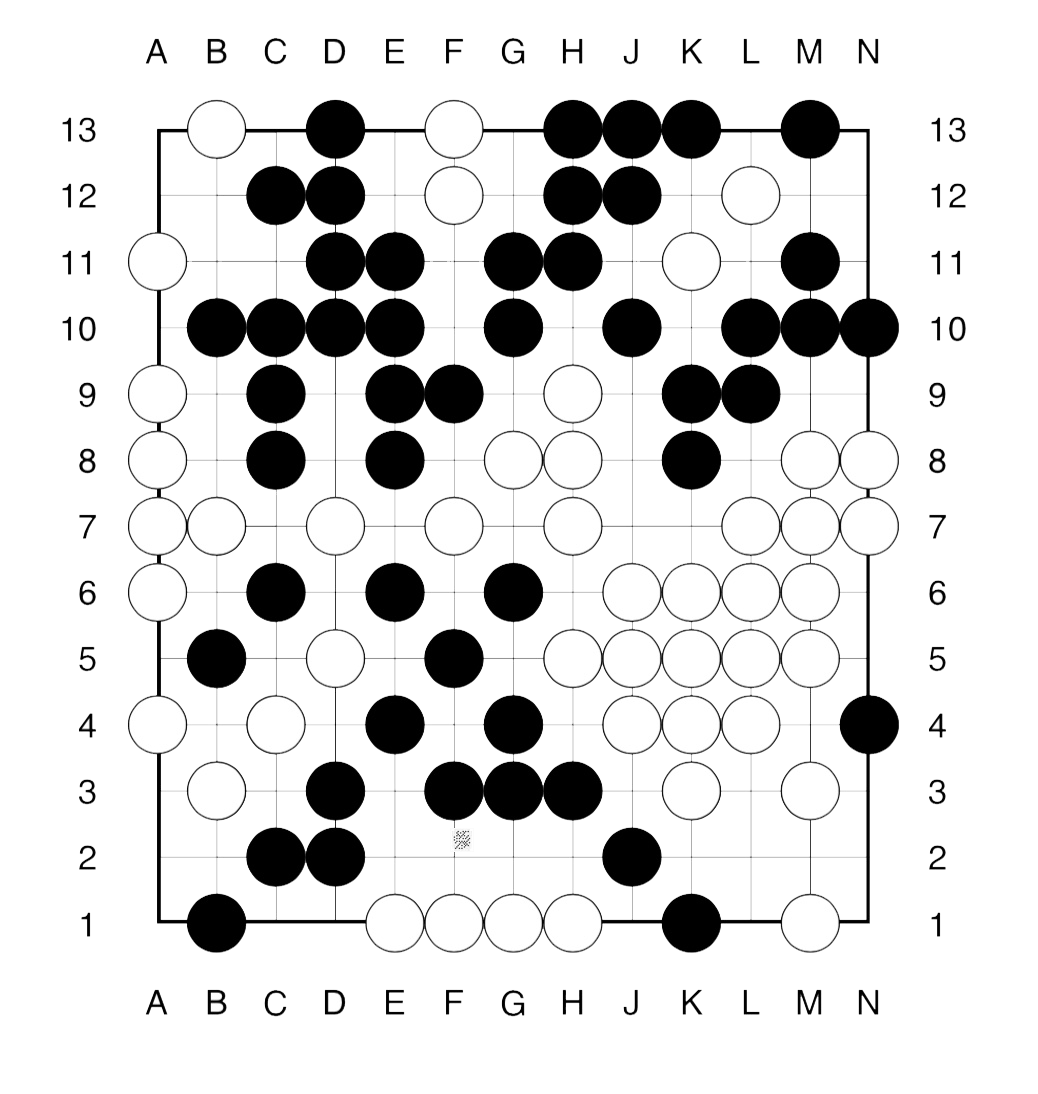The method to approach such an endgame is similar to that in COLgo. First we remove all dead spots from the board.

What remains, are a 4 spots available only to Right (white) and 5 spots available only to Left (bLack). Further, there a 3 ‘live’ regions: the upper righthand corner and the two lower corners.

The value of these corners must be computed inductively.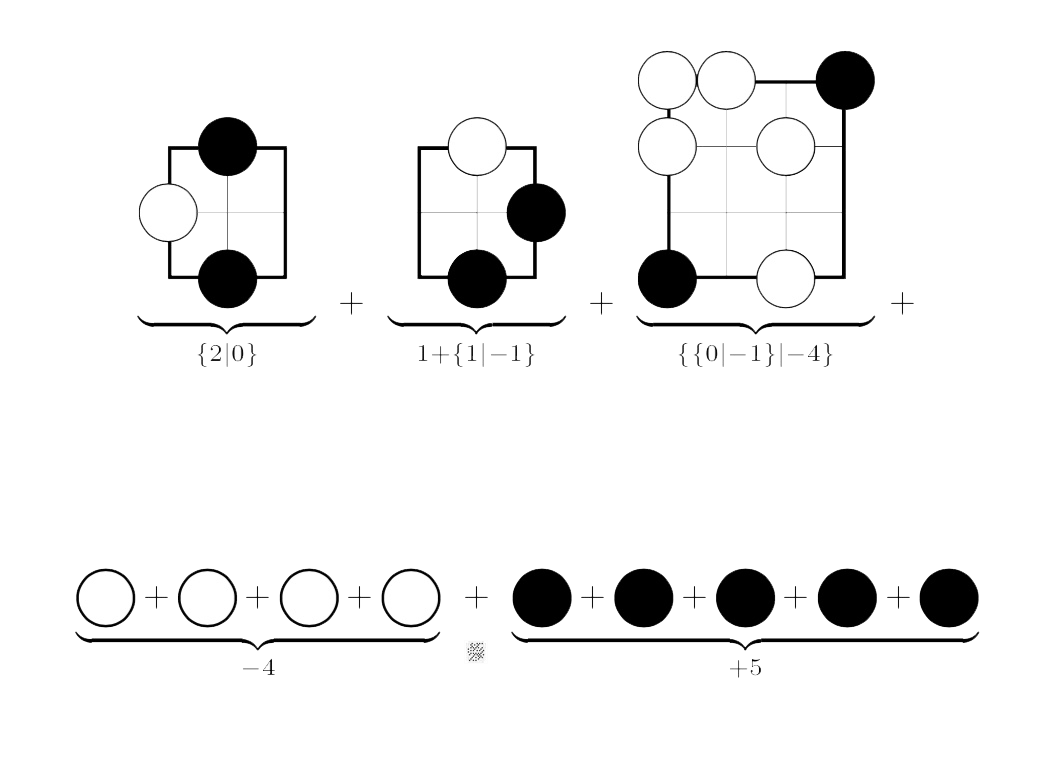For example, Right’s best option in the left-most game (corresponding to the upper righthand corner of the endgame) is to put his stone on N12, resulting in a game in which neither player can move (the zero game).

On the other hand, Left can put a stone at either N11, N12 or N13 leaving a game in which she has two more moves, whereas Right han none (the $2$ game).

The other positions are computed similarly.

To get the value of the endgame we have to sum up all these values.

This can either be done using the addition rule given in ONAG, or by using programs in combinatorial game theory.

There’s Combinatorial Game Suite, developed by Aaron Siegel. But, for some reason I can no longer use it on macOS High Sierra.

Fortunately, the older program David Wolfe’s toolkit is still available, and runs on my MacBook.

The sum game evaluates to $\{ \{3|2 \}|-1 \}$, which is a ‘fuzzy’ game, that is, its value is confused with $0$.

This means that the first player to move has a winning strategy in the endgame.

Can you spot the (unique) winning move for Right (white) and one (of two) winning move for Left (bLack)?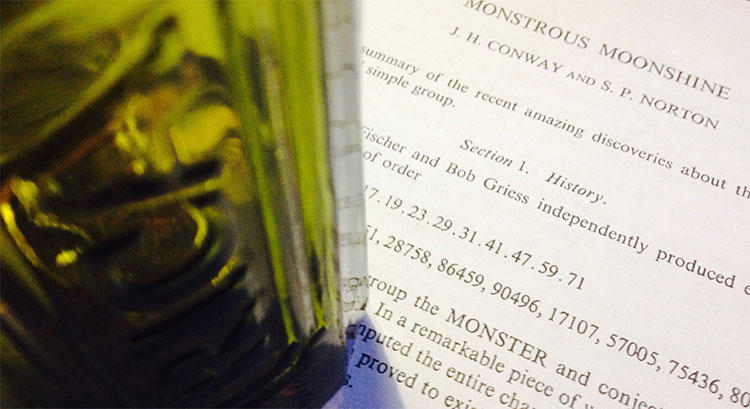At a seminar at the College de France in 1975, Tits wrote down the order of the monster group

$\# \mathbb{M} = 2^{46}.3^{20}.5^9.7^6.11^2.13^3.17·19·23·29·31·41·47·59·71$

Andrew Ogg, who attended the talk, noticed that the prime divisors are precisely the primes $p$ for which the characteristic $p$ super-singular $j$-invariants are all defined over $\mathbb{F}_p$.

Here’s Ogg’s paper on this: Automorphismes de courbes modulaires, Séminaire Delange-Pisot-Poitou. Théorie des nombres, tome 16, no 1 (1974-1975).

Ogg offered a bottle of Jack Daniels for an explanation of this coincidence.

Even Richard Borcherds didn’t claim the bottle of Jack Daniels, though his proof of the monstrous moonshine conjecture is believed to be the best explanation, at present.

A few years ago, John Duncan and Ken Ono posted a paper “The Jack Daniels Problem”, in which they prove that monstrous moonshine implies that if $p$ is not one of Ogg’s primes it cannot be a divisor of $\# \mathbb{M}$. However, the other implication remains mysterious.

Duncan and Ono say:

“This discussion does not prove that every $p ∈ \text{Ogg}$ divides $\# \mathbb{M}$. It merely explains how the first principles of moonshine suggest this implication. Monstrous moonshine is the proof. Does this then provide a completely satisfactory solution to Ogg’s problem? Maybe or maybe not. Perhaps someone will one day furnish a map from the characteristic $p$ supersingular $j$-invariants to elements of order $p$ where the group structure of $\mathbb{M}$ is apparent.”

I don’t know whether they claimed the bottle, anyway.

But then, what is the non-commutative Jack Daniels Problem?

A footnote on the first page of Conway and Norton’s ‘Monstrous Moonshine’ paper says:

“Very recently, A. Pizer has shown these primes are the only ones that satisfy a certain conjecture of Hecke from 1936 relating modular forms of weight $2$ to quaternion algebra theta-series.”

Pizer’s paper is “A note on a conjecture of Hecke”.

Maybe there’s a connection between monstrous moonshine and the arithmetic of integral quaternion algebras. Some hints:

The commutation relations in the Big Picture are reminiscent of the meta-commutation relations for Hurwitz quaternions, originally due to Conway in his booklet on Quaternions and Octonions.

The fact that the $p$-tree in the Big Picture has valency $p+1$ comes from the fact that the Brauer-Severi of $M_2(\mathbb{F}_p)$ is $\mathbb{P}^1_{\mathbb{F}_p}$. In fact, the Big Picture should be related to the Brauer-Severi scheme of $M_2(\mathbb{Z})$.

Then, there’s Jorge Plazas claiming that Connes-Marcolli’s $GL_2$-system might be related to moonshine.

One of the first things I’ll do when I return is to run to the library and get our copy of Shimura’s ‘Introduction to the arithmetic theory of automorphic functions’.

Btw. the bottle in the title image is not a Jack Daniels but the remains of a bottle of Ricard, because I’m still in the French mountains.

Monstrous moonshine associates to every element of order $n$ of the monster group $\mathbb{M}$ an arithmetic group of the form
$(n|h)+e,f,\dots$
where $h$ is a divisor of $24$ and of $n$ and where $e,f,\dots$ are divisors of $\frac{n}{h}$ coprime with its quotient.

In snakes, spines, and all that we’ve constructed the arithmetic group
$\Gamma_0(n|h)+e,f,\dots$
which normalizes $\Gamma_0(N)$ for $N=h.n$. If $h=1$ then this group is the moonshine group $(n|h)+e,f,\dots$, but for $h > 1$ the moonshine group is a specific subgroup of index $h$ in $\Gamma_0(n|h)+e,f,\dots$.

I’m sure one can describe this subgroup explicitly in each case by analysing the action of the finite group $(\Gamma_0(n|h)+e,f,\dots)/\Gamma_0(N)$ on the $(N|1)$-snake. Some examples were worked out by John Duncan in his paper Arithmetic groups and the affine E8 Dynkin diagram.

But at the moment I don’t understand the general construction given by Conway, McKay and Sebbar in On the discrete groups of moonshine. I’m stuck at the last sentence of (2) in section 3. Nothing a copy of Charles Ferenbaugh Ph. D. thesis cannot fix.

The correspondence between the conjugacy classes of the Monster and these arithmetic groups takes up 3 pages in Conway & Norton’s Monstrous Moonshine. Here’s the beginning of it.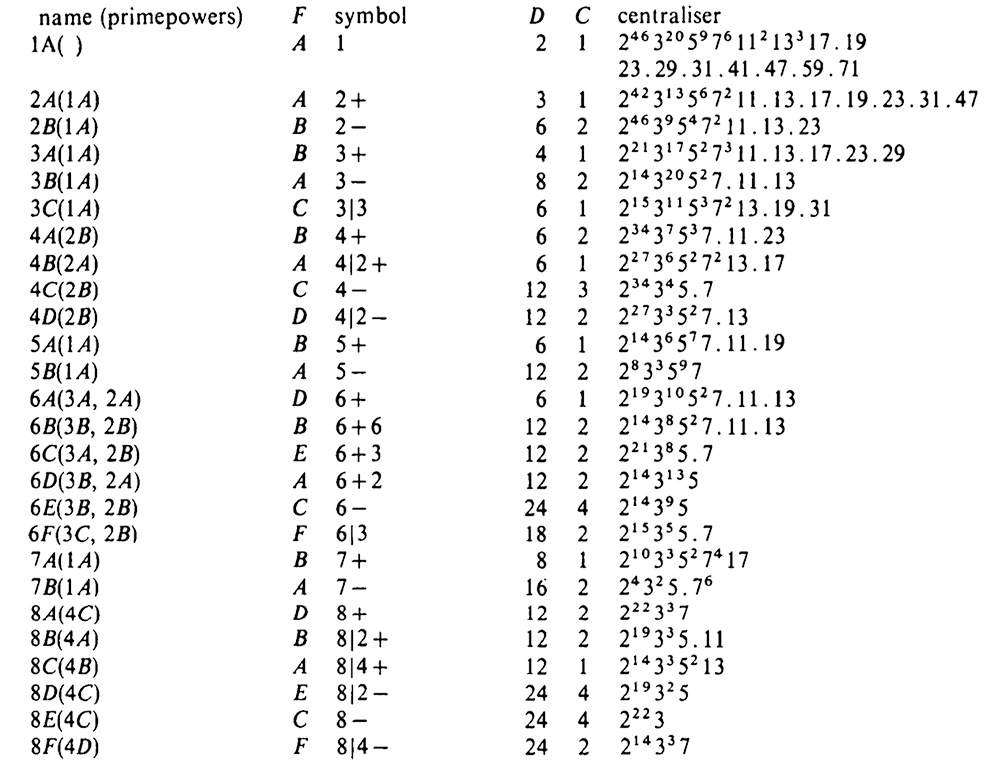From Wikipedia on 24:

“$24$ is the only number whose divisors, namely $1, 2, 3, 4, 6, 8, 12, 24$, are exactly those numbers $n$ for which every invertible element of the commutative ring $\mathbb{Z}/n\mathbb{Z}$ is a square root of $1$. It follows that the multiplicative group $(\mathbb{Z}/24\mathbb{Z})^* = \{ \pm 1, \pm 5, \pm 7, \pm 11 \}$ is isomorphic to the additive group $(\mathbb{Z}/2\mathbb{Z})^3$. This fact plays a role in monstrous moonshine.”

Where did that come from?

In the original “Monstrous Moonshine” paper by John Conway and Simon Norton, section 3 starts with:

“It is a curious fact that the divisors $h$ of $24$ are precisely those numbers $h$ for which $x.y \equiv 1~(mod~h)$ implies $x \equiv y~(mod~h)$.”

and a bit further they even call this fact:

“our ‘defining property of $24$'”.The proof is pretty straightforward.

We want all $h$ such that every unit in $\mathbb{Z}/h \mathbb{Z}$ has order two.

By the Chinese remainder theorem we only have to check this for prime powers dividing $h$.

$5$ is a unit of order $4$ in $\mathbb{Z}/16 \mathbb{Z}$.

$2$ is a unit of order $6$ in $\mathbb{Z}/ 9 \mathbb{Z}$.

A generator of the cyclic group $(\mathbb{Z}/p\mathbb{Z})^*$ is a unit of order $p-1 > 2$ in $\mathbb{Z}/p \mathbb{Z}$, for any prime number $p \geq 5$.

This only leaves those $h$ dividing $2^3.3=24$.

But, what does it have to do with monstrous moonshine?

Moonshine assigns to elements of the Monster group $\mathbb{M}$ a specific subgroup of $SL_2(\mathbb{Q})$ containing a cofinite congruence subgroup

$\Gamma_0(N) = \{ \begin{bmatrix} a & b \\ cN & d \end{bmatrix}~|~a,b,c,d \in \mathbb{Z}, ad-Nbc = 1 \}$

for some natural number $N = h.n$ where $n$ is the order of the monster-element, $h^2$ divides $N$ and … $h$ is a divisor of $24$.

To begin to understand how the defining property of $24$ is relevant in this, take any strictly positive rational number $M$ and any pair of coprime natural numbers $g < h$ and associate to $M \frac{g}{h}$ the matrix $\alpha_{M\frac{g}{h}} = \begin{bmatrix} M & \frac{g}{h} \\ 0 & 1 \end{bmatrix}$ We say that $\Gamma_0(N)$ fixes $M \frac{g}{h}$ if we have that
$\alpha_{M\frac{g}{h}} \Gamma_0(N) \alpha_{M\frac{g}{h}}^{-1} \subset SL_2(\mathbb{Z})$

For those in the know, $M \frac{g}{h}$ stands for the $2$-dimensional integral lattice
$\mathbb{Z} (M \vec{e}_1 + \frac{g}{h} \vec{e}_2) \oplus \mathbb{Z} \vec{e}_2$
and the condition tells that $\Gamma_0(N)$ preserves this lattice under base-change (right-multiplication).

In “Understanding groups like $\Gamma_0(N)$” Conway describes the groups appearing in monstrous moonshine as preserving specific finite sets of these lattices.

For this, it is crucial to determine all $M\frac{g}{h}$ fixed by $\Gamma_0(N)$.

$\alpha_{M\frac{g}{h}}.\begin{bmatrix} 1 & 1 \\ 0 & 1 \end{bmatrix}.\alpha_{M\frac{g}{h}}^{-1} = \begin{bmatrix} 1 & M \\ 0 & 1 \end{bmatrix}$

so we must have that $M$ is a natural number, or that $M\frac{g}{h}$ is a number-like lattice, in Conway-speak.

$\alpha_{M\frac{g}{h}}.\begin{bmatrix} 1 & 0 \\ N & 1 \end{bmatrix}.\alpha_{M\frac{g}{h}}^{-1} = \begin{bmatrix} 1 + \frac{Ng}{Mh} & – \frac{Ng^2}{Mh^2} \\ \frac{N}{M} & 1 – \frac{Ng}{Mh} \end{bmatrix}$

so $M$ divides $N$, $Mh$ divides $Ng$ and $Mh^2$ divides $Ng^2$. As $g$ and $h$ are coprime it follows that $Mh^2$ must divide $N$.

Now, for an arbitrary element of $\Gamma_0(N)$ we have

$\alpha_{M\frac{g}{h}}.\begin{bmatrix} a & b \\ cN & d \end{bmatrix}.\alpha_{M\frac{g}{h}}^{-1} = \begin{bmatrix} a + c \frac{Ng}{Mh} & Mb – c \frac{Ng^2}{Mh^2} – (a-d) \frac{g}{h} \\ c \frac{N}{M} & d – c \frac{Ng}{Mh} \end{bmatrix}$
and using our divisibility requirements it follows that this matrix belongs to $SL_2(\mathbb{Z})$ if $a-d$ is divisible by $h$, that is if $a \equiv d~(mod~h)$.

We know that $ad-Nbc=1$ and that $h$ divides $N$, so $a.d \equiv 1~(mod~h)$, which implies $a \equiv d~(mod~h)$ if $h$ satisfies the defining property of $24$, that is, if $h$ divides $24$.

Concluding, $\Gamma_0(N)$ preserves exactly those lattices $M\frac{g}{h}$ for which
$1~|~M~|~\frac{N}{h^2}~\quad~\text{and}~\quad~h~|~24$

A first step towards figuring out the Moonshine Picture.